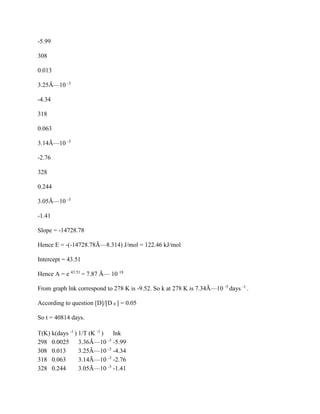Seu SlideShare está sendo baixado. ×

# 2 (12) The following data was obtained for a decomposition of a drug D.docx

Anúncio
Anúncio
Anúncio
Anúncio
Anúncio
Anúncio
Anúncio
Anúncio
Anúncio
Anúncio
AnúncioCarregando em…3
×

1 de 3 Anúncio

# 2 (12) The following data was obtained for a decomposition of a drug D.docx

2 (12) The following data was obtained for a decomposition of a drug D a.)Find the activation energy and A b )If the decomposition follows first order kinetics (In [D]-+In [Do]), how long will it take to decompose by 5% at 5Â°C? T(in Â°C) 25 35 45 /T k in days 0025 013 063 244 Ink
Solution
Arrhenius equation-Â Â Â Â Â  k = A.e -E/RT
K = rate constant
A = pre-exponential factor
E = activation energy
T = temperature
SoÂ Â  lnk = lnA â€“(E/R).(1/T)
Hence a linear fitted plot between lnk vs 1/T will give a straight line with a slope of â€“E/R and intercept lnA.
T(K)
k(days -1 )
1/T (K -1 )
lnk
298
0.0025
3.36Ã—10 -3
-5.99
308
0.013
3.25Ã—10 -3
-4.34
318
0.063
3.14Ã—10 -3
-2.76
328
0.244
3.05Ã—10 -3
-1.41
Slope = -14728.78
Hence E = -(-14728.78Ã—8.314) J/mol = 122.46 kJ/mol
Intercept = 43.51
Hence A = e 43.51 = 7.87 Ã— 10 18
From graph lnk correspond to 278 K is -9.52. So k at 278 K is 7.34Ã—10 -5 days -1 .
According to question [D]/[D 0 ] = 0.05
So t = 40814 days.

.

2 (12) The following data was obtained for a decomposition of a drug D a.)Find the activation energy and A b )If the decomposition follows first order kinetics (In [D]-+In [Do]), how long will it take to decompose by 5% at 5Â°C? T(in Â°C) 25 35 45 /T k in days 0025 013 063 244 Ink
Solution
Arrhenius equation-Â Â Â Â Â  k = A.e -E/RT
K = rate constant
A = pre-exponential factor
E = activation energy
T = temperature
SoÂ Â  lnk = lnA â€“(E/R).(1/T)
Hence a linear fitted plot between lnk vs 1/T will give a straight line with a slope of â€“E/R and intercept lnA.
T(K)
k(days -1 )
1/T (K -1 )
lnk
298
0.0025
3.36Ã—10 -3
-5.99
308
0.013
3.25Ã—10 -3
-4.34
318
0.063
3.14Ã—10 -3
-2.76
328
0.244
3.05Ã—10 -3
-1.41
Slope = -14728.78
Hence E = -(-14728.78Ã—8.314) J/mol = 122.46 kJ/mol
Intercept = 43.51
Hence A = e 43.51 = 7.87 Ã— 10 18
From graph lnk correspond to 278 K is -9.52. So k at 278 K is 7.34Ã—10 -5 days -1 .
According to question [D]/[D 0 ] = 0.05
So t = 40814 days.

.

Anúncio
Anúncio

### 2 (12) The following data was obtained for a decomposition of a drug D.docx

1. 1. 2 (12) The following data was obtained for a decomposition of a drug D a.)Find the activation energy and A b )If the decomposition follows first order kinetics (In [D]-+In [Do]), how long will it take to decompose by 5% at 5Â°C? T(in Â°C) 25 35 45 /T k in days 0025 013 063 244 Ink Solution Arrhenius equation-Â Â Â Â Â k = A.e -E/RT K = rate constant A = pre-exponential factor E = activation energy T = temperature SoÂ Â lnk = lnA â€“(E/R).(1/T) Hence a linear fitted plot between lnk vs 1/T will give a straight line with a slope of â€“E/R and intercept lnA. T(K) k(days -1 ) 1/T (K -1 ) lnk 298 0.0025 3.36Ã—10 -3
2. 2. -5.99 308 0.013 3.25Ã—10 -3 -4.34 318 0.063 3.14Ã—10 -3 -2.76 328 0.244 3.05Ã—10 -3 -1.41 Slope = -14728.78 Hence E = -(-14728.78Ã—8.314) J/mol = 122.46 kJ/mol Intercept = 43.51 Hence A = e 43.51 = 7.87 Ã— 10 18 From graph lnk correspond to 278 K is -9.52. So k at 278 K is 7.34Ã—10 -5 days -1 . According to question [D]/[D 0 ] = 0.05 So t = 40814 days. T(K) k(days -1 ) 1/T (K -1 ) lnk 298 0.0025 3.36Ã—10 -3 -5.99 308 0.013 3.25Ã—10 -3 -4.34 318 0.063 3.14Ã—10 -3 -2.76 328 0.244 3.05Ã—10 -3 -1.41